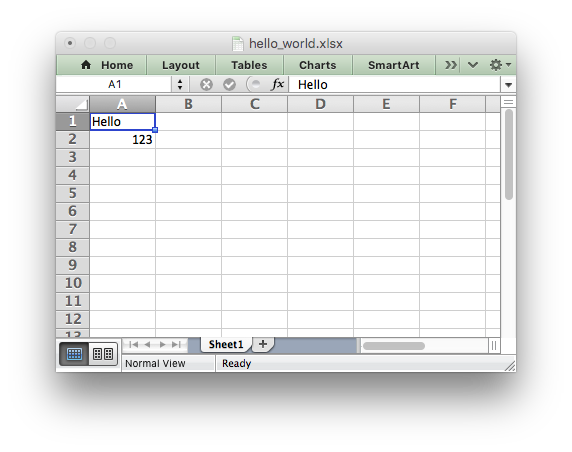libxlsxwriter
hello.c

The simplest possible program and spreadsheet. This is a good place to start to see if the libxlsxwriter library is installed and working correctly./*
* Example of writing some data to a simple Excel file using libxlsxwriter.
*
* Copyright 2014-2021, John McNamara, jmcnamara@cpan.org
*
*/
#include "xlsxwriter.h"
int main() {
lxw_workbook *workbook = workbook_new("hello_world.xlsx");
lxw_worksheet *worksheet = workbook_add_worksheet(workbook, NULL);
worksheet_write_string(worksheet, 0, 0, "Hello", NULL);
worksheet_write_number(worksheet, 1, 0, 123, NULL);
workbook_close(workbook);
return 0;
}
workbook_close
lxw_error workbook_close(lxw_workbook *workbook)
Close the Workbook object and write the XLSX file.
workbook_new
lxw_workbook * workbook_new(const char *filename)
Create a new workbook object.
lxw_worksheet
Struct to represent an Excel worksheet.
Definition: worksheet.h:2107
lxw_workbook
Struct to represent an Excel workbook.
Definition: workbook.h:280
worksheet_write_string
lxw_error worksheet_write_string(lxw_worksheet *worksheet, lxw_row_t row, lxw_col_t col, const char *string, lxw_format *format)
Write a string to a worksheet cell.
worksheet_write_number
lxw_error worksheet_write_number(lxw_worksheet *worksheet, lxw_row_t row, lxw_col_t col, double number, lxw_format *format)
Write a number to a worksheet cell.